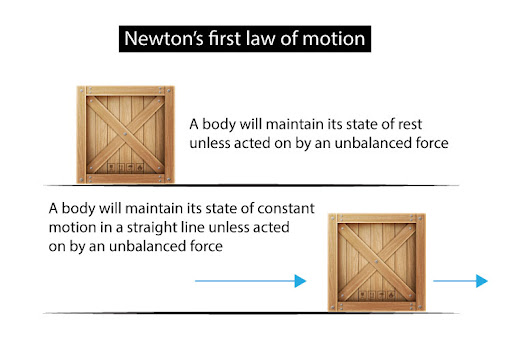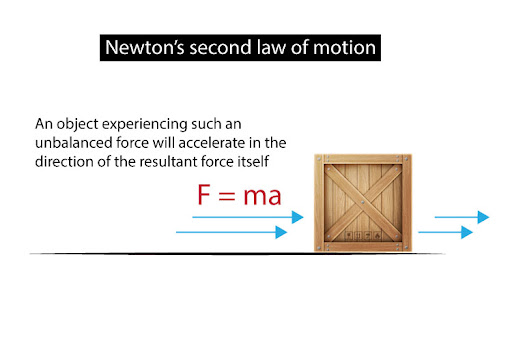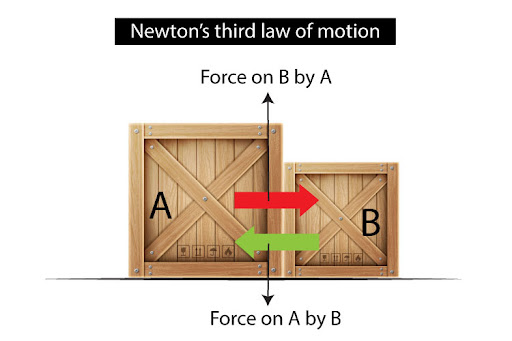# State Newton's 3 Laws in words and/or mathematically

Newton’s first law of motion describes the scenario where no resultant force acts on an object. In such a case, it states that a body will maintain its state of rest or constant motion in a straight line unless acted on by an unbalanced force.This law illustrates an interesting property of matter: a natural tendency to maintain its state of motion, something that we call inertia!

Newton’s second law of motion, then, describes the other scenario: what happens if there is a resultant force acting on an object? Then, the law states that an object experiencing such an unbalanced force will accelerate in the direction of the resultant force itself. Mathematically, it can be written as: F = ma (F = resultant force; m = mass; a  = acceleration)

Newton’s third law of motion is a bit different; it focuses more on the motion of two objects interacting with one another. In that case, it states that when an object A exerts a force on object B, then object B will exert an equal and opposite reaction force back on object A. This illustrates what we call action-reaction pairs, which are forces of the same type with equal magnitude and opposite directions, such that they never act on the same object.